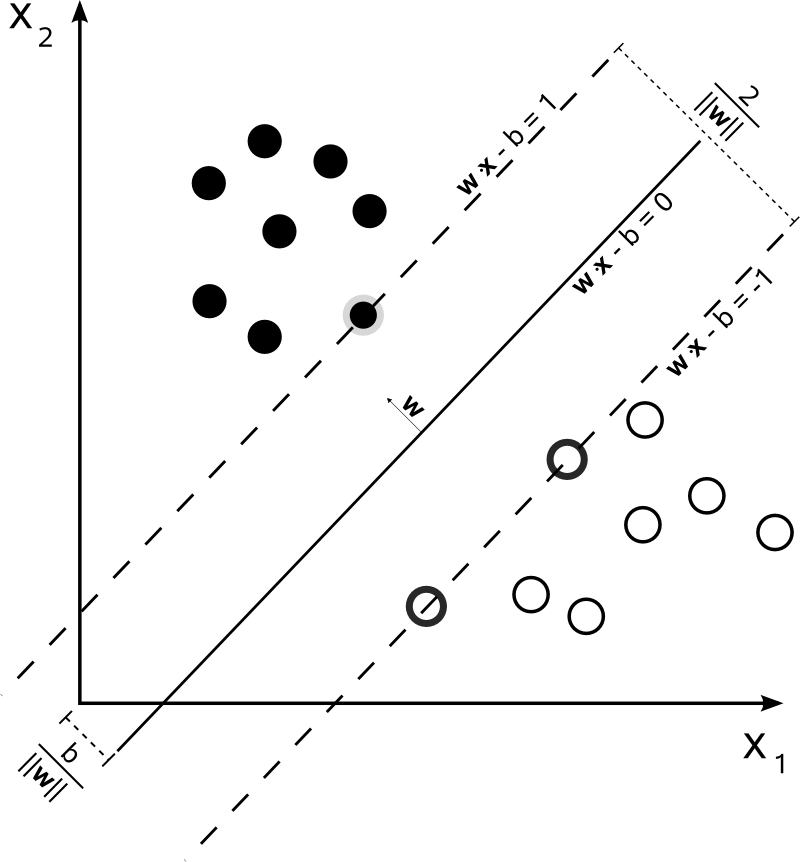# Statistical Learning Theory Learn notes - part II

## Support Vector Machine

· 机器学习,统计学习,算法

1

f(x) = wx + b

• 对所有的正例样本， 有 f(x+) = wx+b > 0,   即 f(x+) * y > 0
• 对所有的负例样本，有f(x-)= wx + b < 0, 即f(x-) * y > 0       (所有负样本 y 的值是 -1）×